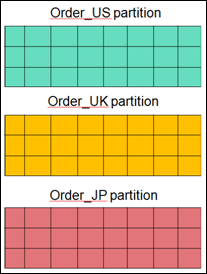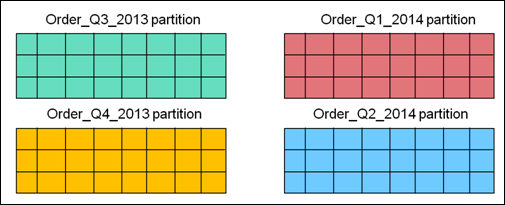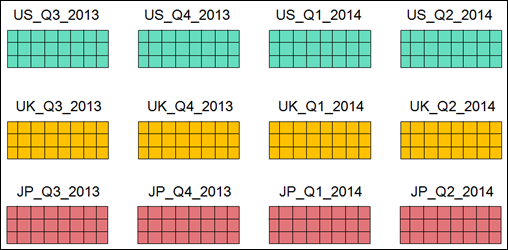Table Partitioning
Introducing Table Partitioning : Table partition types

# Table partition types

Table partitioning enables you to divide the rows of large tables into smaller units, called partitions, and manage each partition individually. The table appears as one single unit to the application, but it is physically divided into multiple partitions in the database. OpenEdge table partitioning supports several different kinds of partitions, as follows:List
For a list partition, the partition definition is based on a single value, and the values for the column that define the partition define a complete set. You cannot create a row that does not match the partition definition.
For example, suppose you had a table of orders containing a country column, and you wanted to partition the column based on the three possible country values, JP, UK and US, the list-partitioned table would look like the following figure:
Figure 1. Example list partitionRange
For a range partition, values for the column that define the partition match particular ranges. Ranges cannot overlap. For example, if you are partitioning based on a date field and defining partitions for a year’s worth of data, your partitions define ranges of January 1 through December 31, for each year.
For example, suppose you had a table of orders containing an order date column, and you wanted to partition the column based on quarters of the calendar year. The range-partitioned table would look like the following figure:
Figure 2. Example range partitionSub-partitions
You can partition a partition, to further refine the subset of a table.List-range — A list-range partition first defines a partition by a list, and then further partitions the data based on a range.List-list — A list-list partition first defines a partition by a list, and then further partitions the data based on a subsequent list.
You can create up to 15 levels of sub-partitions. You can have 15 list sub-partitions, or you can have 14 list sub-partitions, and finally a last sub-partition a range partition.
For example, taking the two previous examples of a list partition and a range partition on a table of orders, combining them into a list-range partitioned table would look like the following figure:
Figure 3. Example list-range partitionComposite
A composite partition is a partition that contains the data for multiple partition definitions in the same physical partition. Intended as a migration tool, when initially partitioning an existing table, all data resides in the composite initial partition.